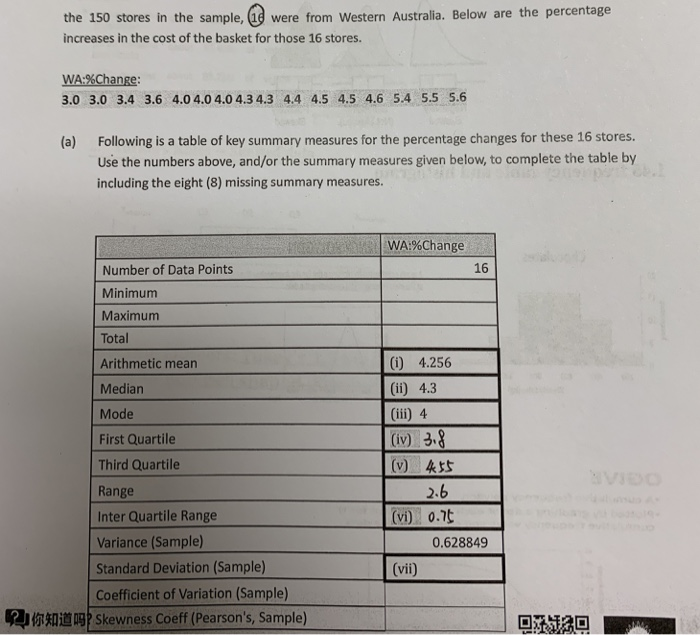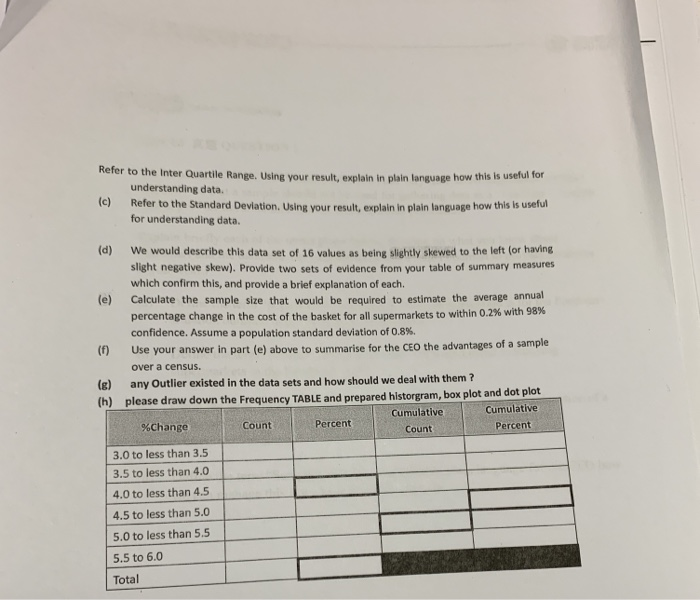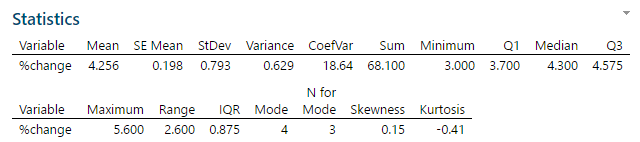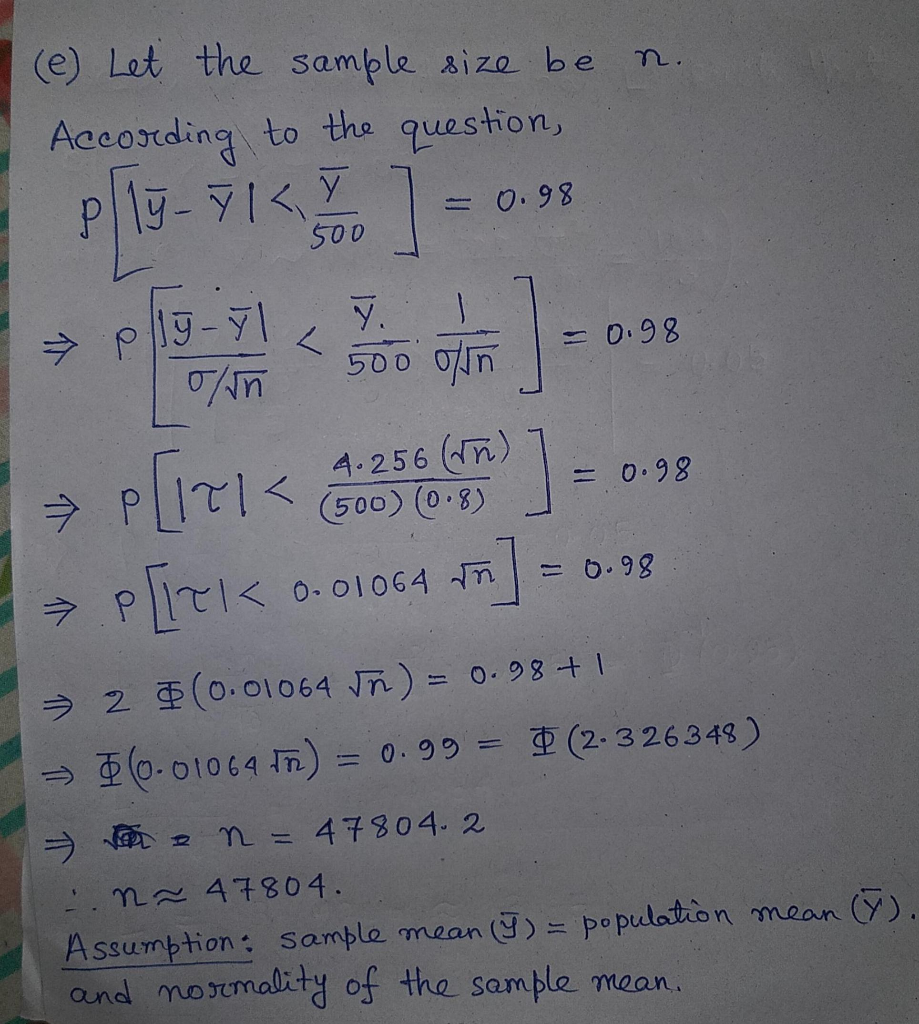# the 150 stores in the sample, (19 were from Western Australia. Below are the percentage increases in the cost of t...the 150 stores in the sample, (19 were from Western Australia. Below are the percentage increases in the cost of the basket for those 16 stores WA %Change 3.0 3.0 3.4 3.6 4.0 4.0 4.0 4.3 4.3 44 4.5 4.5 4.6 5.4 5.5 5.6 (a) Following is a table of key summary measures for the percentage changes for these 16 stores. Use the numbers above, and/or the summary measures given below, to complete the table by including the eight (8) missing summary measures WA%Change 16 Number of Data Points Minimum Maximum Total (i) 4.256 Arithmetic mean (ii) 4.3 Median Mode 3-8 First Quartile (iv) Third Quartile Range Inter Quartile Range (vi) 0.t Variance (Sample) 0.628849 Standard Deviation (Sample) (vii) Coefficient of Variation (Sample) @你知道吗 Skewness Coeff (Pearson's, Sample)
Refer to the Inter Quartile Range. Using your result, explain in plain language how this is useful for understanding data. (c) Refer to the Standard Devlation. Using your result, explain in plain language how this is useful for understanding data (d) We would describe this data set of 16 values as being slightly skewed to the left (or having slight negative skew). Provide two sets of evidence from your table of summary measures which confirm this, and provide a brief explanation of each. (e) Calculate the sample size that would be required to estimate the average annual percentage change in the cost of the basket for all supermarkets to within 0.2% with 98% confidence. Assume a population standard deviation of 0.8%. () Use your answer in part (e) above to summarise for the CEO the advantages of a sa over a census. (8) any Outlier existed in the data sets and how should we deal with them ? please draw down the Frequency TABLE and prepared historgram, box plot and dot plot Cumulative Cumulative Percent Percent %Change Count Count 3.0 to less than 3.5 3.5 to less than 4.0 4.0 to less than 4.5 4.5 to less than 5.0 5.0 to less than 5.5 5.5 to 6.0 Total

Here I am using Minitab 18.

(a)(b) Interquartile Range (IQR) is measure of dispersion of a data set. It measures the spread of the distribution of the data set.

If a data point lies below (Q1-1.5*IQR) and above (Q2+1.5*IQR), then the data point would be considered as an outlier.

(c) Standard Deviation is also a measure of dispersion. From the given data set the SD is 0.793, which means that on an average all the data points are deviated from the mean by 0.793

(d) The skewness is 0.15, indicating moderately positively skewed. It means that right tail is longer (frequency curve). Also we have noticed that mean < median which is typically occurred for a negative skew distribution. So, overall we say that the distribution is more or less symmetric.

(e)##### Add Answer of: the 150 stores in the sample, (19 were from Western Australia. Below are the percentage increases in the cost of t...
More Homework Help Questions Additional questions in this topic.